# The Basic Relational Model and Relational Algebra Introduction

• Slides: 12
Download presentation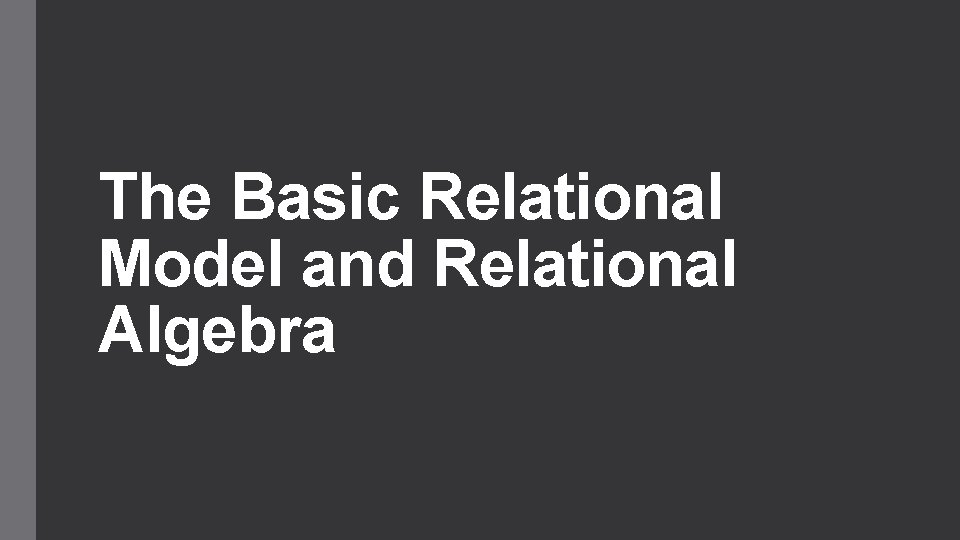The Basic Relational Model and Relational AlgebraIntroduction • The relational data model was first introduced by Ted Codd of IBM Research in 1970 • The model uses the concept of a mathematical relation—which looks somewhat like a table of values —as its basic building block, and has its theoretical basis in set theory and first-order predicate logic • The first commercial implementations of the relational model became available in the early 1980 • Relational Model Concepts Relational Model represents the database as collection of relations Relation is thought of as a table of values Flat Files Table has – Table Name and Column Names, from which we can interpret the meaning of the values Relation STUDENT USN NAME EMAIL ID CONTACT No 01 FMXXMCAXX [email protected] XX XXXXX Attribute Tuple Domains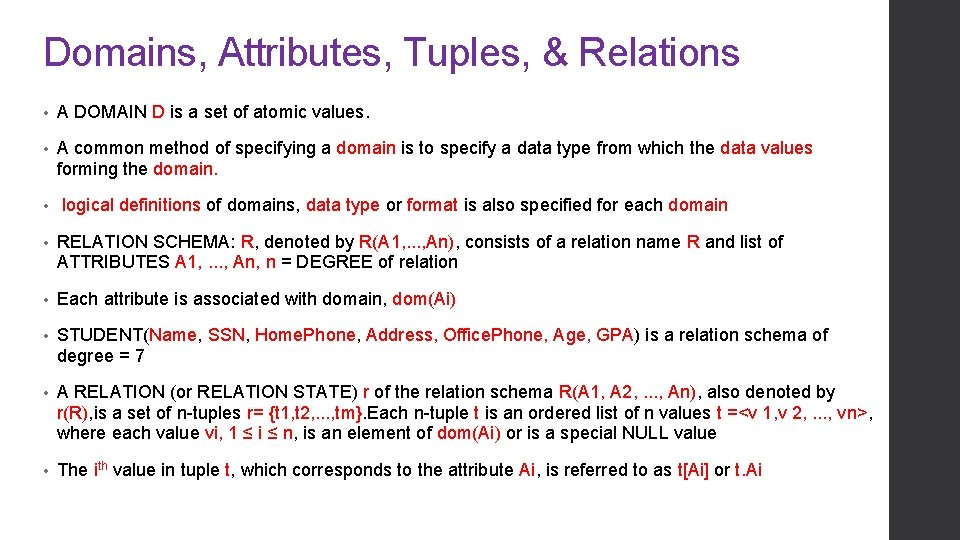Domains, Attributes, Tuples, & Relations • A DOMAIN D is a set of atomic values. • A common method of specifying a domain is to specify a data type from which the data values forming the domain. • logical definitions of domains, data type or format is also specified for each domain • RELATION SCHEMA: R, denoted by R(A 1, . . . , An), consists of a relation name R and list of ATTRIBUTES A 1, . . . , An, n = DEGREE of relation • Each attribute is associated with domain, dom(Ai) • STUDENT(Name, SSN, Home. Phone, Address, Office. Phone, Age, GPA) is a relation schema of degree = 7 • A RELATION (or RELATION STATE) r of the relation schema R(A 1, A 2, . . . , An), also denoted by r(R), is a set of n-tuples r= {t 1, t 2, . . . , tm}. Each n-tuple t is an ordered list of n values t =<v 1, v 2, . . . , vn>, where each value vi, 1 ≤ i ≤ n, is an element of dom(Ai) or is a special NULL value • The ith value in tuple t, which corresponds to the attribute Ai, is referred to as t[Ai] or t. Ai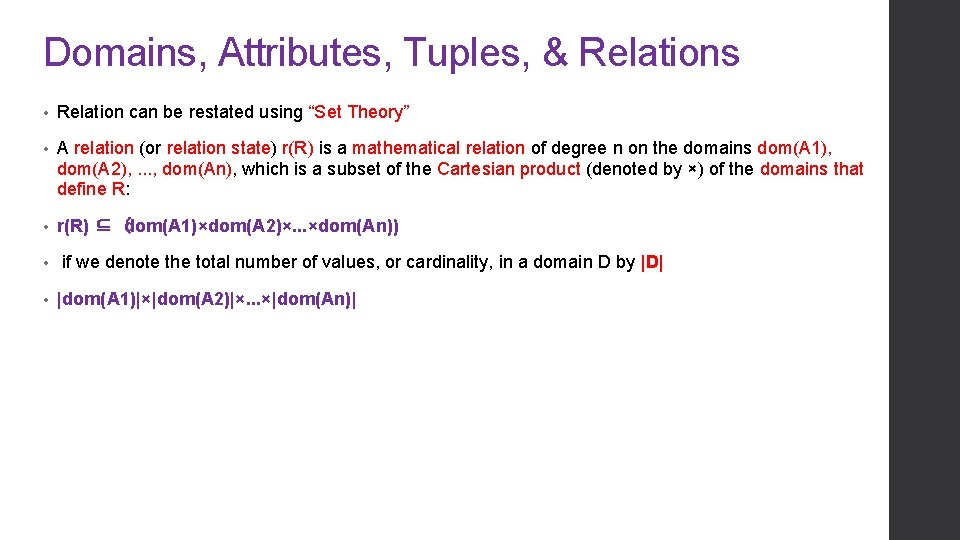Domains, Attributes, Tuples, & Relations • Relation can be restated using “Set Theory” • A relation (or relation state) r(R) is a mathematical relation of degree n on the domains dom(A 1), dom(A 2), . . . , dom(An), which is a subset of the Cartesian product (denoted by ×) of the domains that define R: • r(R) ⊆ (dom(A 1)×dom(A 2)×. . . ×dom(An)) • • if we denote the total number of values, or cardinality, in a domain D by |D| |dom(A 1)|×|dom(A 2)|×. . . ×|dom(An)|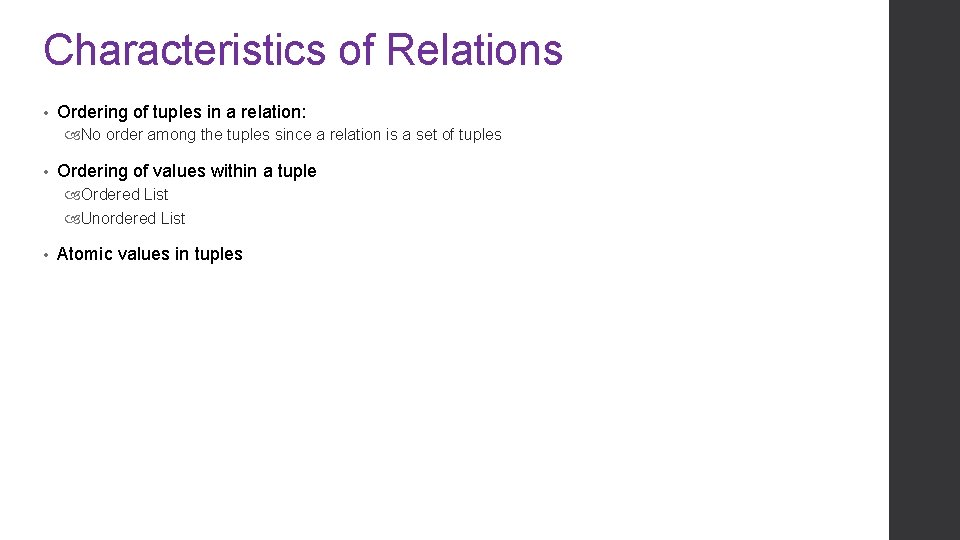Characteristics of Relations • Ordering of tuples in a relation: No order among the tuples since a relation is a set of tuples • Ordering of values within a tuple Ordered List Unordered List • Atomic values in tuples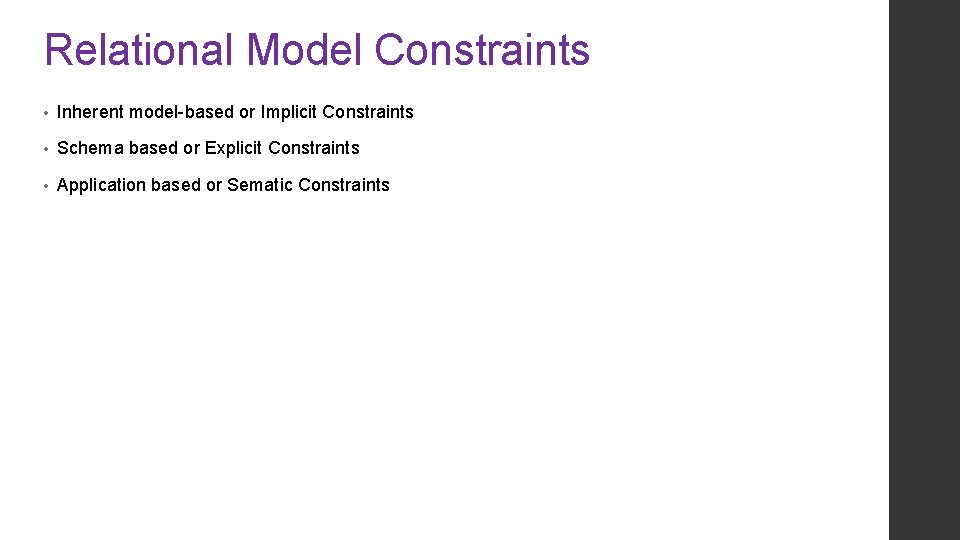Relational Model Constraints • Inherent model-based or Implicit Constraints • Schema based or Explicit Constraints • Application based or Sematic Constraints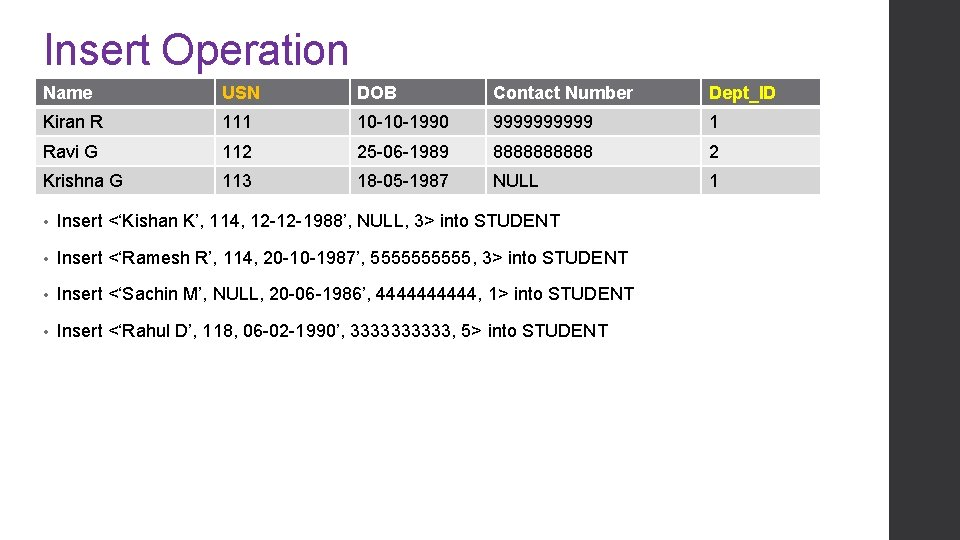Insert Operation Name USN DOB Contact Number Dept_ID Kiran R 111 10 -10 -1990 99999 1 Ravi G 112 25 -06 -1989 88888 2 Krishna G 113 18 -05 -1987 NULL 1 • Insert <‘Kishan K’, 114, 12 -12 -1988’, NULL, 3> into STUDENT • Insert <‘Ramesh R’, 114, 20 -10 -1987’, 55555, 3> into STUDENT • Insert <‘Sachin M’, NULL, 20 -06 -1986’, 44444, 1> into STUDENT • Insert <‘Rahul D’, 118, 06 -02 -1990’, 33333, 5> into STUDENT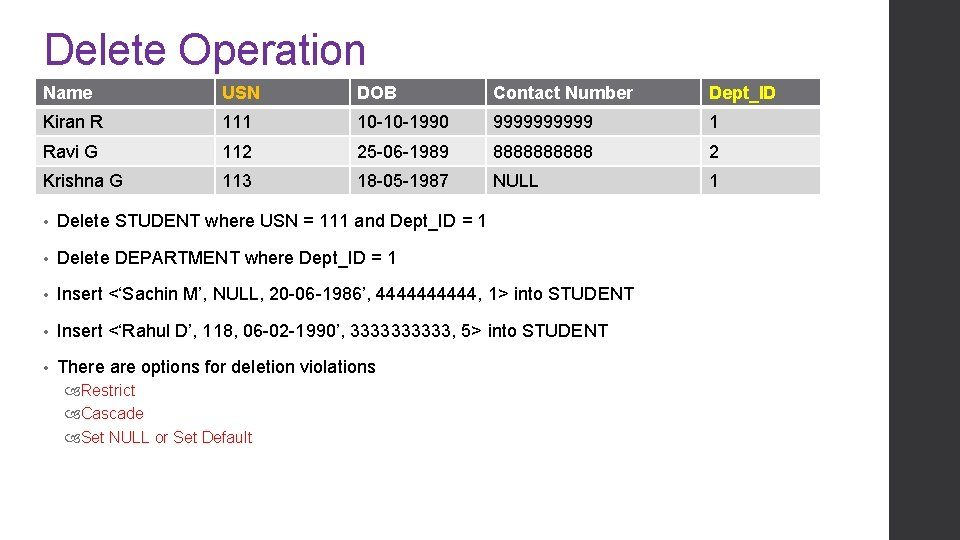Delete Operation Name USN DOB Contact Number Dept_ID Kiran R 111 10 -10 -1990 99999 1 Ravi G 112 25 -06 -1989 88888 2 Krishna G 113 18 -05 -1987 NULL 1 • Delete STUDENT where USN = 111 and Dept_ID = 1 • Delete DEPARTMENT where Dept_ID = 1 • Insert <‘Sachin M’, NULL, 20 -06 -1986’, 44444, 1> into STUDENT • Insert <‘Rahul D’, 118, 06 -02 -1990’, 33333, 5> into STUDENT • There are options for deletion violations Restrict Cascade Set NULL or Set DefaultUpdate Operation Name USN DOB Contact Number Dept_ID Kiran R 111 10 -10 -1990 99999 1 Ravi G 112 25 -06 -1989 88888 2 Krishna G 113 18 -05 -1987 NULL 1 • UPDATE DOB of STUDENT USN=111 to 12 -10 -1990 • UPDATE Dept_ID of STUDENT USN=111 to 5 • UPDATE USN of STUDENT USN=111 to 113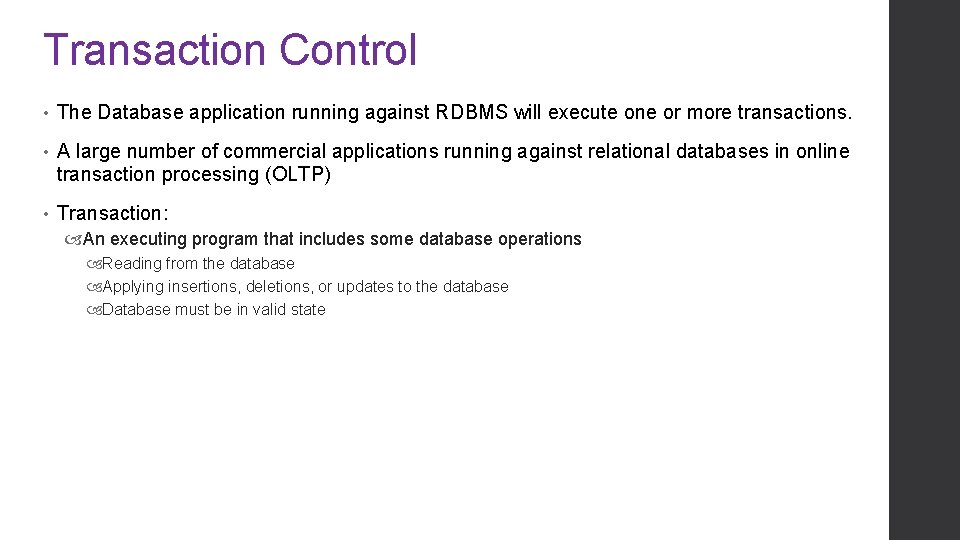Transaction Control • The Database application running against RDBMS will execute one or more transactions. • A large number of commercial applications running against relational databases in online transaction processing (OLTP) • Transaction: An executing program that includes some database operations Reading from the database Applying insertions, deletions, or updates to the database Database must be in valid state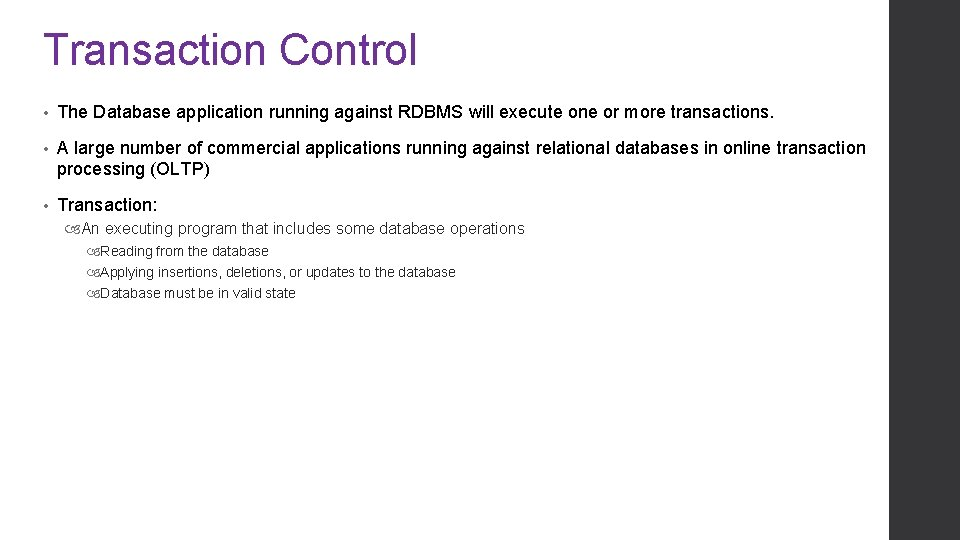Transaction Control • The Database application running against RDBMS will execute one or more transactions. • A large number of commercial applications running against relational databases in online transaction processing (OLTP) • Transaction: An executing program that includes some database operations Reading from the database Applying insertions, deletions, or updates to the database Database must be in valid stateThank You!!!